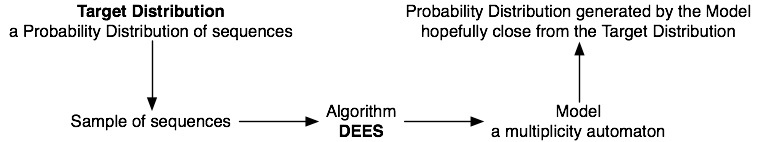- Sytle -
Blue . Blob . LIF . Grey . Red!

# Research

## DEES

What is DEES and what it does? Because a good picture is better than a long speech...I had developped an implementation of DEES in C++. This is a multiplicity automata inference algorithm. This program does around 7,500 lines (10,000 if comments are counted)

This is a machine learning program. It take a sample of many sequences (or words) generated by a target probability distribution and return a model (a multiplicity automaton) generating a probability distribution as close as possible of the target distribution.

We can restric the learned model to be:

• a Probabilistic Automaton (PA) (another name for Hidden Markov Models - HMM) ; in this case the identified class is the set of Probabilistic Residual Automata (PRA)
• a Probabilistic Deterministic Automaton (PDA)

### Features

The main features of DEES are:

• Multiplicity automata (MA) inference from a sample of sequences.
• Probabilistic Automata (PA) Inference
• Probabilistic Deterministic Automata (PDA) Inference

DEES has also another features:

• Baulm-Welch algorithm
• Random generation methods of MA, PA, PRA and PDA
• GraphViz export of models
• Sample generation from an MA
• Model class detection (MA, PA, PRA, PDA)
• Calculus (if the value exists) ofwhere A is the name of an MA.
• convertion between Alergia, MDI and DEES file format ; sample and automata
• Generation of the trimmed MA of an MA in linear time
• If GraphViz is intalled, model are shown and export them in PDF

If you wish to use DEES send me an email.

DEES uses a library under the GPL, its use is then restricted.

A version without this dependency exists but is far less efficient. This is why I don't give a direct link for DEES, but prefer to be asked for.

DEES was done for experiment theoretical algorithms. It never been programmed to touch many people (there is no UI for example).

Entirely done with Vim
Validation: [xhtml] . [css]